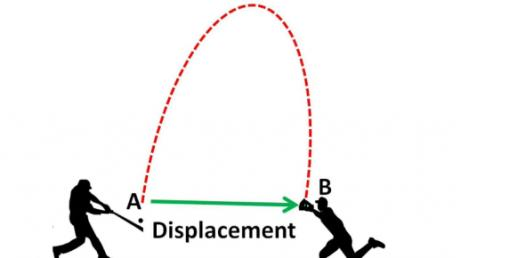# Quiz: Can You Pass The Distance And Displacement Test?

7 Questions | Attempts: 435
ShareSettingsCan You Pass The Distance And Displacement Test? When you exert the right amount of force on an object, it is bound to take motion, and the distance the original position to where it starts is the displacement distance. Take up this trivia quiz and get to see if you can figure out the distance an object moves. All the best!

• 1.
What is the total displacement of a student who walks 3 blocks east, 2 blocks north, 1 block west, and then 2 blocks south?
• A.

8 blocks

• B.

0

• C.

2 blocks east

• D.

2 block west

• 2.
Which two terms represent a vector quantity and the scalar quantity of the vector's magnitude, respectively?
• A.

Weight and force

• B.

Speed and time

• C.

Displacement and distance

• D.

Acceleration and velocity

• 3.
A girl leaves a history classroom and walks 10. meters north to a drinking fountain. Then she turns and walks 30. meters south to an art classroom. What is the girl's total displacement from the history classroom to the art classroom?
• A.

40 m north

• B.

20 m north

• C.

20 m south

• D.

40 m south

• 4.
A student on her way to school walks four blocks east, three blocks north, and another four blocks east, as shown in the diagram. Compared to the distance she walks, the magnitude of her displacement from home to school is
• A.

Less

• B.

Greater

• C.

The same

• D.

Can't be determined

• 5.
The map shows the route traveled by a school bus. What is the magnitude of the total displacement of the school bus from the start to the end of its trip?
• A.

800 m

• B.

400m

• C.

1800m

• D.

500m

• 6.
A softball player leaves the batter's box, overruns first base by 3.0 meters, and then returns to first base. Compared to the total distance traveled by the player, the magnitude of the player's total displacement from the batter's box is
• A.

Smaller

• B.

Can't be determined

• C.

The same

• D.

Larger

• 7.
What is the total displacement of a student who walks 3 blocks east, 2 blocks north, 1 block west, and then 2 blocks south?
• A.

8 blocks

• B.

0

• C.

2 blocks west

• D.

2 blocks east

## Related TopicsBack to top
×

Wait!
Here's an interesting quiz for you.